# Extending dataReporter

## Introduction

The data documentation tools available in `dataReporter` allows for it to be utilized as an easy-to-use, self-contained package, as outlined in our article. However, this does not imply that `dataReporter` is not customizable: `dataReporter` was specifically built for including user-made extensions and this vignette is a tutorial into how one can write simple extensions that can be used in e.g. the `makeDataReport()` function from `dataReporter`.

### Three steps of data documentation

In order to understand how different parts of the report creation can be customized, we will briefly dwell on the structure of the data reports made by `dataReporter`. Aside from a few pages dedicated to gaining an overview of the full dataset and what was done to document its current state, the report sequentially presents information for each variable. More specifically, each variable is presented with one of the two sets of information:

1. The result of a single eligibility check (referred to as a pre-check below)
2. The results of the three canonical `dataReporter` steps:
• Summarize: Produces a summary table
• Visualize: Produces a distribution visualization
• Check: Performs and lists the results of a number of checks

The first option will be used for variables that do not pass the initial pre-checks. These are checks that are performed in order to identify variables that are not eligible for detailed documentation for one reason or another. The default pre-checks used in `makeDataReport()` are

• `isKey`: Check if the variable is a key, i.e. categorical with unique values for each observation
• `isSingular`: Check if the variable only takes a single (non-`NA`) value
• `isSupported`: Check if the class of the variable is among the classes supported by `dataReporter`

If any of these checks finds a problem, the relevant problem will be mentioned in the report and the variable will not be exposed to further documentation steps. If the pre-checks do not flag a variable, on the other hand, the variable will be subjected to the three SVC (summarize, visualize, check) steps mentioned above. In the SVC-steps, `makeDataReport()` calls a number of so-called `summaryFunctions`, `visualFunctions` and `checkFunctions`, with different choices of functions depending on the class of the variable. The customizability features of `dataReporter` essentially comes down to writing such `summaryFunctions`, `visualFunctions` and `checkFunctions`.

This vignette consists of three parts. First, we describe how new `summaryFunction`s, `visualFunction`s and `checkFunctions` can be made by describing the requirements for their structures that must be fulfilled in order for them to be used in a `makeDataReport()` call. Secondly, we turn to a worked example of how to write custom made functions in practice. Here, we define and showcase six new SVC functions. Lastly, a built-in dataset about art masterpieces, `artData`, is documented using the custom-made functions and the thereby obtained data report is included.

In this vignette, we focus on the report creation tools of `dataReporter`. However, it should be emphasized that by following the guidelines presented here, the user-defined `summaryFunctions`, `visualFunctions` and `checkFunctions` will also be fully integrated with the interactive mode of `dataReporter`.

## Function templates

In order to construct a summary, visual or check function, one needs to create a new function with a specific structure. This can be done with different levels of strictness. If the new custom function is only to be used as part of the SVC steps in `makeDataReport()`, then only the input/output structure of the function needs special attention. However, new user-defined functions can also be registered locally to be part of the full machinery of `dataReporter`, and when this is done, the functions will be recognized and behave in the same way as the built-in functions in `dataReporter`. The presentation below is given in the format of function templates, written in pseudo-code. These templates are designed for getting the full functionality, but note that the table below serves as a reference to the minimal requirements for each function type, while also presenting the “full” versions.

For each of the three function types, we have provided an S3 object class. This was done in order to facilitate obtaining overviews of all available options for summaries, visuals and checks through calls to the three functions `allSummaryFunctions()`, `allVisualFunctions()` and `allCheckFunctions()`. Similarly, we have also provided specific output classes for the summary- and check outputs, for which convenient printing methods are available.

This table summarizes the minimal and recommended structures for summary-, visual- and check functions:

`summaryFunction` `visualFunction` `checkFunction`
Input (required) `v` - A variable vector.
`...` - Additional arguments passed to the function.
`v` - A variable vector.
`vnam` - The variable name (as character string).
`doEval` - A logical (`TRUE`/`FALSE`) controlling the output type of the function.
`v` - A variable vector
`nMax` - An integer (or `Inf`), controlling how many problematic values are printed, if relevant
`...` - Additional arguments passed to the function.
Input (optional) `maxDecimals` - The number of decimals printed in outputted numerical values. `maxDecimals` - The number of decimals printed in outputted numerical values.
Purpose Describe some aspect of the variable, e.g., a central value, its dispersion or level of missingness. Produce a distribution plot. Check a variable for a specific issue and, if relevant, identify the values in the variable that cause the issue.
Output (required) A list with entries:
`feature` - A label for the summary value (as character string);
`result` - The result of the summary (as character string).
A character string with `R` code for producing a plot. This code should be standalone, i.e., should include the data if necessary. A list with entries:
`problem` - A logical identifying whether an issue was found;
`message` - A character string (possibly empty) describing the issue that was found, properly escaped and ready for use with `rmarkdown`.
Output (recommended) A `summaryResult` object, i.e., an attributed list with entries `feature`, `result` and `value`, the latter being the values from `result` in their original format). If `doEval` is `TRUE`: A plot that is opened by the graphic device in `R`.
If `doEval` is `FALSE`: A text string with `R` code, as described above.
A `checkResult` object, i.e., an attributed list with entries `problem`, `message` and `problemValues`, the latter being either `NULL` or the problem causing values, as they were found in `v`, whichever is relevant.
Tools available for producing the function `summaryResult()` `messageGenerator()`
`checkResult()`

### Writing a summaryFunction

As mentioned above, `dataReporter` provides a dedicated class for `summaryFunction`s. However, this does not imply that they are particularly advanced or complicated to create; in fact, they are nothing but regular functions with a particular input/output-structure. Specifically, they all follow the template below:

``````mySummaryFunction <- function(v, ...) {
val <- [result of whatever summary we are doing]
res <- [properly escaped version of val]
summaryResult(list(feature = "[Feature name]", result = res,
value = val))
}``````

The last function called here, `summaryResult()`, changes the class of the output, thereby making a `print()` method available for it. Note that `v` is an input vector and that `res` should be either a character string or something that will be printed as one. In other words an integer would be allowed for `res`, but a matrix will not. Note that, strictly speaking, only one of the two elements `value` and `result` is needed in order to create a `summaryResult`. If `result` is provided, this will be printed as the summary result. However, if only `value` is provided, the `summaryResult()` function will try to convert it to a character string itself. This might be more or less difficult to do in a reasonable way, so therefore, we really do recommend to provide this conversion yourself by both returning a `result` and a `value`.

Though a lot of different things can go into the `summaryFunction` template, we recommend only using it for summarizing the features of a variable, and leaving tests and checks for the `checkFunctions` (presented below).

Adhering to the template above is sufficient for using the freshly made `mySummaryFunction()` in `makeDataReport()`, but we recommend adding the new function to the overview of all summary functions by converting it to a proper `summaryFunction` object. This is done by calling the `summaryFunction()` creator with the user-defined function as the first argument, and additional arguments `description` (an explanatory text which will be added to the attributes of the function), and `classes` (a vector of variable classes the user-defined function is intended to be applied to, also stored as an attribute). In other words, a call following the template below should be made:

``````mySummaryFunction <- summaryFunction(mySummaryFunction,
description = "[Text describing what the summaryFunction does]",
classes = c([vector of data types that the function is intended
for]))``````

which adds the new function to the output of an `allSummaryFunctions()` call. If `mySummaryFunction` is constructed as an `S3` generic function with associated methods, the call to `summaryFunction()` will automatically produce a vector of the names of the classes for which the function can be called. If `mySummaryFunction()` is not an `S3` generic and `classes` is left unspecified, the attribute will simply be left empty. Note that the helper function `allClasses()` might be useful for filling out the `classes` argument, as it simply lists all supported classes in `dataReporter`:

``allClasses()``
``````##  "character"      "Date"           "factor"         "integer"
##  "labelled"       "haven_labelled" "logical"        "numeric"``````

### Writing a visualFunction

`visualFunction`s are the functions that produce the figures in a `dataReporter` output document. Writing a `visualFunction` is slightly more complicated than writing a `summaryFunction`. This is due to the fact that `visualFunction`s need to be able to output standalone code for plots in order for `makeDataReport()` to build standalone `rmarkdown` files. We recommend using the following structure (again shown as pseudo code):

``````myVisualFunction <- function(v, vnam, doEval) {
thisCall <- call("[the name of the function used to produce the plot]",
v, [additional arguments for the plotting function])
if (doEval) {
return(eval(thisCall))
} else return(deparse(thisCall))
}``````

In this function, `v` is the variable to be visualized, `vnam` is its name (which should generally be passed to `title` or `main` arguments in plotting functions) and `doEval` controls whether the output is a plot (if `TRUE`) or a character string of standalone code for producing a plot (if `FALSE`). Implementing the `doEval = TRUE` setting is not strictly necessary for a `visualFunction`’s use in `makeDataReport()`, but it makes it easier to assess what visualization options are available, and obviously, it is crucial for interactive usage of `myVisualFunction()`. In either case, it should be noted that all the parameters listed above, `v`, `vnam` and `doEval`, are mandatory, so they must be left as is, even if they are not in use (c.f., the table).

As with the summary function, we call `visualFunction()` to register our newly created function:

``````myVisualFunction <- visualFunction(myVisualFunction,
description = "[Some text describing the visualFunction]",
classes = c([data types that this function is intended for]))
)``````

Now, `myVisualFunction()` will be available in a `allVisualFunctions()` call, just like the two build-in `visualFunctions`, `standardVisual` and `basicVisual`.

### Writing a checkFunction

The last, but perhaps most important, `dataReporter` function type is the `checkFunction`. These are the functions that flag issues in the data in the check step and/or control the overall flow of the data documenting process in the pre-check stage. A `checkFunction` follows one of two overall structures, depending on the type of check. Either, it tries to identify problematic values in the variable (as e.g., `identifyMissing()` does), or it performs a check concerning the variable as a whole (e.g., the functions used for pre-checks and the function `identifyNums()`). We present templates for both types of `checkFunction`s below separately, but it should be emphasized that formally, they belong to the same class.

First, a template for the full-variable check function type, where we first define the function and subsequently register it as a check function using `checkFunction()`:

``````myFullVarCheckFunction <- function(v, ...) {
[do your check]
problem <- [is there a problem? TRUE/FALSE]
message <- "[message describing the problem, if any]"
checkResult(list(problem = problem,
message = message,
problemValues = NULL))
}

myFullVarCheckFunction <- checkFunction(myFullVarCheckFunction,
description = "[Some text describing the checkFunction]",
classes = c([the data types that this function is intended to be used for])
)``````

Again, as with `summaryFunctions` and `visualFunctions`, the change of function class by use of `checkFunction()` is not strictly necessary. Note however, that if `myFullVarCheckFunction` is to be used in the summarize/visualize/check steps in `makeDataReport()`, the description attribute will be printed in the overview table in the Data report overview part of the report.

If problematic values are to be identified, the template from above should be expanded to follow a slightly more complicated structure (again shown as pseudo-code):

``````myProbValCheckFunction <- function(v, nMax, maxDecimals, ...) {
[do your check]
problem <- [is there a problem? TRUE/FALSE]
problemValues <- [vector of values in v that are problematic]
problemStatus <- list(problem = problem,
problemValues = problemValues)

problemMessage <- "[Message that is printed prior to listing
problem values in the dataReporter output,
ending with a colon]"

outMessage <- messageGenerator(problemStatus, problemMessage, nMax)

checkResult(list(problem = problem,
message = outMessage,
problemValues = problemValues))
}

myProbValCheckFunction <- checkFunction(myProbValCheckFunction,
description = "[Some text describing the checkFunction]",
classes = c([the data types that this function is intended to be used for])
)``````

One comment should be devoted to the helper function, `messageGenerator()`. This function’s sole purpose is aiding consistent styling of all `checkFunction` messages. The function simply pastes together the `problemMessage` and the `problemValues`, with the latter being quoted and sorted alphabetically. If the `nMax` argument to `messageGenerator()` is not `Inf`, only the first `nMax` problem values will be pasted onto the message, accompanied by a comment about how many problem values were left out (if any). Note that printing quotes in rmarkdown requires an extensive amount of character escaping, so opting for `messageGenerator()` really is the easiest solution.

In the template above, the argument `maxDecimals` is not in use. This argument should be used to round off the `problemValues` passed to `messageGenerator()`, if they are numerical. This can be done by adding an extra line of code after defining `problemStatus` in the template above:

``````myProbValCheckFunction <- function(v, nMax, maxDecimals, ...) {
[... more lines of code here ...]
problemStatus <- list(problem = problem,
problemValues = problemValues)

if (!is.null(problemValues)) {
problemStatus\$problemValues <- round(problemValues, maxDecimals)
}
[... more lines of code here ...]
}``````

Now, problematic values will be rounded in the outputted message, while they will still appear in their original format under the entry `problemValues` in the returned object.

## A worked example

As an example, we now create some new functions and show both how they can be used interactively and how they can be integrated with the `makeDataReport()` function. These new functions are:

• New `summaryFunctions`:
• `countZeros()`: A new `summaryFunction` that counts the number of occurrences of the value `0` in a variable. This function will be used in the summarize step of `makeDataReport()`.
• `meanSummary()`: A new `summaryFunction` that computes the mean of numerical variables. This function is also intended to be used in the summarize step.
• New `visualFunctions`:
• `mosaicVisual()`: A new `visualFunction` that produces mosaic plots. This function will be used in the visualize step of `makeDataReport()`.
• `prettierHist()`: A new `visualFunction` that makes `ggplot2` histograms, but with contours around each bar (as is the default in the `graphics` histograms). This function will also be used in the visualize step.
• New `checkFunctions`:
• `isID()`: A new `checkFunction` intended for use in the pre-check-stage. This function checks whether a variable consists exclusively of long (at least 8 characters/digits) entries that are all of equal length, as this might be personal identification codes that we do not wish to print out in the data summary.
• `identifyColons()` A new `checkFunction` that flags variables in which some observations have colons that appear in between other characters. This is practical for identifying autogenerated interaction effects. This function will be used in the check step of `makeDataReport()`.

These functions are defined in turn below, and afterwards, an example of how they can be called from `makeDataReport()` is provided.

### summaryFunction examples: countZeros() and meanSummary()

We start by defining two summary functions. For both functions, we use `summaryResult()` for creating the function output and then we use `summaryFunction()` in order to add the functions to the output from a `allSummaryFunctions()` call.

#### countZeros — a simple summaryFunction

First, we will make a summary function that counts how many times the value `0` occurs in a variable - either as a character or as a numeric value. We define this `summaryFunction` in the following lines of code:

``````countZeros <- function(v, ...) {
val <- length(which(v == 0))
summaryResult(list(feature = "No. zeros", result = val, value = val))
}``````

As this function computes an integer (the number of zeros), there is no difference between the elements `\$result` and `\$value`. If, on the other hand, the result had been a character string, extra formatting might be required in the `\$result` entry (such as escaping of quotation marks), and in this scenario, the two entries would have differed.

Because the result is returned as a `summaryResult` object, a printing method is automatically called when `countZeros()` is used interactively:

``````#Called on a numeric variable
countZeros(c(rep(0, 5), 1:100))``````
``## No. zeros: 5``
``````#Called on a character variable
countZeros(c(rep(0, 5), letters))``````
``## No. zeros: 5``

Note that `letters` is a globally defined vector consisting of all the letters in the (English) alphabet as characters.

We change the class of `countZeros()` in order to make it appear in `allSummaryFunctions()` calls. Moreover, we wish to emphasize that the function is not intended to be called on all variable types, as zeros have different roles in `Date` and `logical` variables:

``````countZeros <- summaryFunction(countZeros,
description = "Count number of zeros",
classes = c("character", "factor", "integer",
"labelled", "numeric"))``````

Please note that this is just meta-information: The function can still be called on `Date` and `logical` variables, but then the user will know that they are acting against the recommendations of the programmer. In order to control what input variables can actually be used, we suggest writing the function as an S3 generic with methods for some, but not all, data classes, as the next example will show.

#### meanSummary — an S3 generic summary function

In this example, we will use the concept of method dispatch and generic S3 functions in order to control what data classes a `summaryFunction` can be used for. If you are not familiar with S3 classes, we refer to Hadley Wickham’s Advanced R for a excellent, though somewhat computer science heavy, introduction to object oriented programming in R.

We would like to define a very simple summary function that computes the arithmetic mean of a variable. This is of course only meaningful for numerical variables, i.e. variables with the classes numeric`,`integer`or`logical`. Therefore, we will use the S3 framework to force users to only call our function on these appropriate classes.

First, we define the generic function:

``````meanSummary <- function(v, maxDecimals = 2) {
UseMethod("meanSummary")
}``````

This is just an empty shell: All it does is to say that if the function `meanSummary` is called, R should look for class-specific methods called `meanSummary`. However, no such methods are defined yet, and therefore an error is produced if the function is called:

``meanSummary(1)``
``## Error in UseMethod("meanSummary"): no applicable method for 'meanSummary' applied to an object of class "c('double', 'numeric')"``

We now define a helper function that input a variable `v`, removes `NA`s, computes the mean and outputs this mean as a proper `summaryResult`:

``````meanSummaryHelper <- function(v, maxDecimals) {
#remove missing observations
v <- na.omit(v)

#compute mean and store "raw" output in `val`
val <- mean(v)

#store printable (rounded) output in `res`
res <- round(val, maxDecimals)

#output summaryResult
summaryResult(list(feature = "Mean", result = res, value  = val))
}``````

and we then assign this function as the `meanSummary` method used for `logical`, `numeric` and `integer` variables:

``````#logical
meanSummary.logical <- function(v, maxDecimals = 2) {
meanSummaryHelper(v, maxDecimals)
}

#numeric
meanSummary.numeric <- function(v, maxDecimals = 2) {
meanSummaryHelper(v, maxDecimals)
}

#integer
meanSummary.integer <- function(v, maxDecimals = 2) {
meanSummaryHelper(v, maxDecimals)
}``````

and now we can see what happens when the function is called on supported - and non-supported - variables:

``````#called on a numeric variable (supported)
meanSummary(rnorm(100))``````
``## Mean: 0.03``
``````#called on a character variable - produces error as there is
#no method for characters
meanSummary(letters)``````
``## Error in UseMethod("meanSummary"): no applicable method for 'meanSummary' applied to an object of class "character"``

Finally, we will make it a proper `summaryFunction`. Because we defined `meanSummary()` as an S3 generic function, we do not have to specify which classes it should be called up - `summmaryFunction()` will automatically look up what classes have methods and are thus supported:

``````meanSummary <- summaryFunction(meanSummary,
description = "Compute arithmetic mean")``````

And now it appears - together with `countZeros` - in a `allSummaryFunctions()` call:

``allSummaryFunctions()``
name description classes
countZeros Count number of zeros character, factor, integer, labelled, numeric
meanSummary Compute arithmetic mean integer, logical, numeric
centralValue Compute median for numeric variables, mode for categorical variables character, Date, factor, integer, labelled, haven_labelled, logical, numeric
countMissing Compute proportion of missing observations character, Date, factor, integer, labelled, haven_labelled, logical, numeric
minMax Find minimum and maximum values integer, numeric, Date
quartiles Compute 1st and 3rd quartiles Date, integer, numeric
refCat Find reference level factor
uniqueValues Count number of unique values character, Date, factor, integer, labelled, haven_labelled, logical, numeric
variableType Data class of variable character, Date, factor, integer, labelled, haven_labelled, logical, numeric

### visualFunction examples: mosaicVisual() and prettierHist()

We will now define two different visual functions. The first one is a `graphics`-based function, while the second one is `ggplot2`-based.

#### mosaicVisual — a new visualFunction for categorical data

In the base `R` package `graphics`, a function which produces mosaic plots is available. It is intended to be called on one-way tables, as. e.g. returned from `table()`. This function is named `mosiacplot` and results in the following type of plots:

``````#construct a character variable by sampling 100 values that are
#either "a" (probability 0.3) or "b" (probability 0.7):
x <- sample(c("a", "b"), size = 100, replace = TRUE,
prob = c(0.3, 0.7))

#draw a mosaic plot of the distribution:
mosaicplot(table(x))``````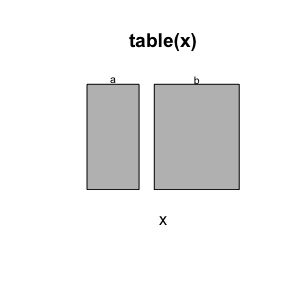As evident from the figure, a mosaic plot consists of several rectangles, one for each category in the data, and the area of each such rectangle is proportional to the proportion of the data that takes that value - much like a pie chart. We intend to use this function as part of a new visualization function. We will define the new function such that it gets the full `dataReporter` functionality. This can be done using the following code which sets up the call using the existing function `mosaicplot()`.

``````mosaicVisual <- function(v, vnam, doEval) {
#Define a (unevaluated) call to mosaicplot
thisCall <- call("mosaicplot", table(v), main = vnam, xlab = "")

#if doEval is TRUE, evaluate the call, thereby producing a plot
#if doEval is FALSE, return the deparsed call
if (doEval) {
return(eval(thisCall))
} else return(deparse(thisCall))
}``````

This function can now be called directly or used in `makeDataReport()`, as will presented in an example below. Depending on the `doEval` argument, either a text string with code or a plot is produced. We can for instance inspect the code for the plot produced in the above by calling `mosaicVisual()` on `x` with `doEval = FALSE`:

``mosaicVisual(x, "Variable x", doEval = FALSE)``
``````##  "mosaicplot(structure(c(a = 38L, b = 62L), .Dim = 2L, .Dimnames = list("
##  "    v = c(\"a\", \"b\")), class = \"table\"), main = \"Variable x\", "
##  "    xlab = \"\")"``````

Even though `mosaicVisual()`, as written above, follows the style of a `visualFunction`, it is not yet truly one and therefore, it will not appear in an `allVisualFunctions()` call. In order to get this functionality, we need to change its object class. This can be done by writing

``````mosaicVisual <- visualFunction(mosaicVisual,
description = "Mosaic plots using graphics",
classes = setdiff(allClasses(),
c("numeric",
"integer",
"Date")))``````

Here, we use the function `allClasses()` to quickly obtain a vector of all the seven variable classes supported by `dataReporter`, and we use `setdiff()` to choose all classes except the `numeric`, `integer` and `Date` classes, as the mosaic plot is most suited for categorical data. Note that if `mosaicVisual()` were an S3 generic function, this argument could have been left as `NULL` and then the classes for which methods are available would be added automatically by `visualFunction()`.

#### prettierHist() — a customized ggplot2 histogram

Next, we will define a `ggplot2`-based plotting function. We will simply make a slight alteration of the looks of the typical `ggplot2` histogram, but it serves of a general example of how `ggplot2` visual functions can be built.

But first, we define a helper function that does the actual plotting. This makes it simpler to write a visual function using the `call()`/`eval()` structure presented in the above, thereby making it easy to provide a visual function that can both be used interactively (with `doEval = TRUE`) and in `makeDataReport()` (with `doEval = FALSE`). The helper function is defined like this:

``````library(ggplot2)

prettierHistHelper <- function(v, vnam) {
#define a ggplot2 histogram
p <- ggplot(data.frame(v = v), aes(x = v)) +
geom_histogram(col = "white", bins = 20) +
xlab(vnam)

#return the plot
p
}``````

We use `col = "white"` to add white contours around the bars in the histogram. Let’s look at an example of this function called on a variable consisting of 100 random draws from the standard normal distribution:

``prettierHistHelper(rnorm(100), "Standard normal variable")``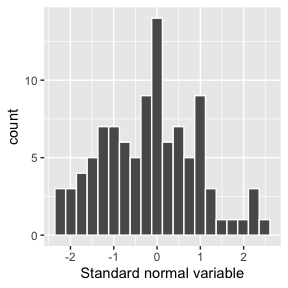Now, we can define a visual function that calls `prettierHistHelper()`:

``````#define visualFunction-style prettierHist()-function
prettierHist <- function(v, vnam, doEval = TRUE) {
#define the call
thisCall <- call("prettierHistHelper", v = v, vnam = vnam)

#evaluate or deparse
if (doEval) {
return(eval(thisCall))
} else return(deparse(thisCall))
}

#Make it a proper visualFunction:
prettierHist <- visualFunction(prettierHist,
description = "ggplot2 style histogram with contours",
classes = c("numeric", "integer", "logical", "Date"))``````

We specify that the `prettierHist()` should only be called on variables of the classes `numeric`, `integer`, `logical` or `Date`, as is reasonable for a histogram. And we will now find our new `visualFunctions` in a `allVisualFunctions()`-call:

``allVisualFunctions()``
name description classes
mosaicVisual Mosaic plots using graphics character, factor, labelled, haven_labelled, logical
prettierHist ggplot2 style histogram with contours numeric, integer, logical, Date
basicVisual Histograms and barplots using graphics character, Date, factor, integer, labelled, haven_labelled, logical, numeric
standardVisual Histograms and barplots using ggplot2 character, Date, factor, integer, labelled, haven_labelled, logical, numeric
tableVisual Distribution tables character, factor, labelled, haven_labelled

### checkFunction examples: isID() and identifyColons()

Next up is two new check functions. First, we will define a check function that is intended for use in the pre-check stage, i.e. used for screening variables for eligibility for the summarize/visualize/check-steps. This will be a function that checks the entries in a variable for a certain structure which often used in ID type variables and we will name the new function `isID()`. Afterwards, we will define a check function that looks for `:` in categorical variables, as these might be the result of unintended interactions introduced in the data wrangling process.

#### isID — a new checkFunction without problem values

First, let’s define the `isID()` function. As this function is not supposed to list problematic values, it falls within the category of `checkFunctions` represented by `myFullVarCheckFunction()` above. We do not particularly wish to use this function interactively, so we will stick to the minimal requirements of a `checkFunction` used in `check()` (see the overview table in the beginning of this vignette). We are interested in checking whether variables that are neither `logical` nor `Date` are restricted to a certain structure. In this example, we simply check if the all observations, when converted to characters, have the same lengths and that length is at least 8. This might be regarded as minimal restrictions for a lot of different ID type information, including US social security numbers and credit card numbers, and of course, a more specific ID check function can be built as an expansion of `isID()`. We define the function:

``````isID <- function(v, nMax = NULL, ...) {
#define minimal output. Note that this is not a
#proper checkResult
out <- list(problem = FALSE, message = "")

#only perform check if the variable is neither a logical nor a Date
if (class(v) %in% setdiff(allClasses(), c("logical", "Date"))) {

#count the number of characters in each entry of v
v <- as.character(v)
lengths <- c(nchar(v))

#check if all entries of v have at least 8 characters
#and whether they all have the same length. If so,
#flag as a problem.
if (all(lengths >= 8) & length(unique(lengths)) == 1) {
out\$problem <- TRUE
out\$message <- "Warning: This variable seems to contain ID codes."
}
}

#return result of the check
out
}``````

This is essentially all we need to do in order to include this function as a pre-check-function in `makeDataReport()`. However, we should note that e.g. not using `checkResult()` for the output means that it does not have a convenient `print()` method available; now, the output just really is displayed as a list:

``````#define 9-character ID variable:
idVar <- c("1234-1233", "9221-0289",
"9831-1201", "6722-1243")

#check for ID resemblance for the ID variable
isID(idVar)``````
``````## \$problem
##  TRUE
##
## \$message
##  "Warning: This variable seems to contain ID codes."``````
``````#check for ID resemblance for a non-ID variable
isID(rnorm(10))``````
``````## \$problem
##  FALSE
##
## \$message
##  ""``````

As we have not changed the class of this check function to be a `checkFunction`, it should be noted that it will not show up in the output of a `allCheckFunctions()` call.

#### identifyColons — a new checkFunction with problem values

The last function we will define in this vignette is `identifyColons()`. We define it using the helper function `messageGenerator()` to obtain a properly escaped message, and we use `checkResult()` to make its output print neatly. As mentioned above, the purpose of this check function is to identify variables where colons appear, as they might have been introduced by mistake when loading or wrangling the data. In the code we use regular expressions through the `gregexpr()` function to identify colons that appear in between other characters:

``````identifyColons <- function(v, nMax = Inf, ... ) {
#remove duplicates (for speed) and missing values:
v <- unique(na.omit(v))

#Define the message displayed if a problem is found:
problemMessage <- "Note that the following values include colons:"

#Initialize the problem indicator (`problem`) and
#the faulty values (`problemValues`)
problem <- FALSE
problemValues <- NULL

#Identify values in v that has the structure: First something (.),
#then a colon (:), and then something again (.), i.e. values with
#non-trailing colons:
problemValues <- v[sapply(gregexpr(".:.", v),
function(x) all(x != -1))]

#If any problem values are identified, set the problem indicator
#accordingly
if (length(problemValues) > 0) {
problem <- TRUE
}

#Combine the problem indicator and the problem values
#into a problem status object that can be passed to
#the messageGenerator() helper function that will
#make sure the outputted message is properly escaped
#for inclusion in the dataReporter report
problemStatus <- list(problem = problem,
problemValues = problemValues)
outMessage <- messageGenerator(problemStatus, problemMessage, nMax)

#Output a checkResult with the problem, the escaped
#message and the raw problem values.
checkResult(list(problem = problem,
message = outMessage,
problemValues = problemValues))
}``````

And we change the class of the function so that it becomes a proper `checkFunction` object:

``````identifyColons <- checkFunction(identifyColons,
description = "Identify non-trailing colons",
classes = c("character", "factor", "labelled"))``````

Note, that for `checkFunctions`, the description provided in a `checkFunction()` call will appear in the report produced by `makeDataReport()` (in the Data cleaning summary section), so for this type of function, the change of class is not only done for the sake of the `allCheckFunctions()` output.

Let’s see the results of `identifyColons()` when used interactively on a potentially problematic variable:

``````#define a variable as an interaction between between two factors:
iaVar <- factor(c("a", "b", "a", "c")):factor(c(1, 2, 3, 4))

#Check iaVar for colons:
identifyColons(iaVar)``````
``## Note that the following values include colons: a:1, a:3, b:2, c:4.``

And when used on a variable that does not contain colons:

``identifyColons(letters)``
``## No problems found.``

### Calling the new summarize/visualize/check functions from makeDataReport()

Now, we are ready to use all the new functions in a `makeDataReport()` call. We wish to produce a report that utilizes the newly defined functions in the following way:

• We want to add the new pre-check function, `isID()`, to the already existing pre-checks.
• We wish to change the plot type for `factor` variables to be `mosaicVisual()`, while `numeric`, `integer` and `Date` variables should use `prettierHist()` for their visualizations.
• We want the new summary function, `countZeros()`, to be added to the summaries performed on all variable types except for `Date` and `logical`.
• We want the new summary function, `meanSummary()`, to be used for `numeric`, `integer` and `logical` variables.
• We want the new check function, `identifyColons()`, to be added to the checks performed on `character`, `factor` and `labelled` variables.

We will make a data report for the built-in dataset `artData` that is based on the Master Works of Art data from Data Explorer. The dataset contains 200 observations (corresponding to paintings) of 11 variables, describing different properties of the paintings and their artists. The first 5 observations look like this:

``````data(artData)
head(artData, 5)``````
``````##   ArtistID              ArtistName NoOfMiddlenames
## 1 29da884a          Georges Seurat               0
## 2 f055cbb8           Giacomo Balla               0
## 3 5323b3b8 Lucas Cranach the Elder               2
## 4 ca07b695       Hugo van der Goes               2
## 5 02161eeb              Grant Wood               0
##                                                 Title Year
## 1 A Sunday Afternoon on the Island of La Grande Jatte 1886
## 2                            Abstract Speed and Sound 1914
## 3                            Adam and Eve in Paradise 1531
## 4                              Adoration of the Kings 1470
## 5                                     American Gothic 1930
##                   Location     Continent  Width Height     Media
## 1 Art Institute of Chicago North America 308.00 207.60 oil paint
## 2        Guggenheim Museum North America  76.52  54.61 oil paint
## 3           Gemaldegalerie        Europe  35.50  50.40 oil paint
## 4           Gemaldegalerie        Europe 242.00 147.00 oil paint
## 5 Art Institute of Chicago North America  62.40  74.30 oil paint
##                                           Movement
## 1 Post-Impressionist:Neo-Impressionist:Pointillist
## 2                                         Futurism
## 3                               German Renaissance
## 4                             Northern Renaissance
## 5                                      Regionalist``````

The 11 variables of `artData` can be summarized as follows:

• `ArtistID`: A unique ID used for cataloging the artists (fictional)
• `ArtistName`: The name of the artist
• `NoOfMiddleNames`: The number of middle names the artist has
• `Title`: The title of the painting
• `Year`: The approximate year in which the painting was made
• `Location`: The current location of the painting
• `Continent`: The continent of the current location of the painting
• `Width`: The width of the painting, in centimeters
• `Height`: The height of the painting, in centimeters
• `Media`: The media/materials of the painting
• `Movement`: The artistic movement(s) the painting belongs to

The options for the data report described above are specified as follows:

``````makeDataReport(artData,
#add extra precheck function
preChecks = c("isKey", "isSingular", "isSupported", "isID"),

#Add the extra summaries - countZeros() for character, factor,
#integer, labelled and numeric variables and meanSummary() for integer,
#numeric and logical variables:
summaries = setSummaries(
character = defaultCharacterSummaries(add = "countZeros"),
factor = defaultFactorSummaries(add = "countZeros"),
labelled = defaultLabelledSummaries(add = "countZeros"),
numeric = defaultNumericSummaries(add = c("countZeros", "meanSummary")),
integer = defaultIntegerSummaries(add = c("countZeros", "meanSummary")),
logical = defaultLogicalSummaries(add =  c("meanSummary"))
),

#choose mosaicVisual() for categorical variables,
#prettierHist() for all others:
visuals = setVisuals(
factor = "mosaicVisual",
numeric = "prettierHist",
integer = "prettierHist",
Date = "prettierHist"
),

#Add the new checkFunction, identifyColons, for character, factor and
#labelled variables:
checks = setChecks(
character = defaultCharacterChecks(add = "identifyColons"),
factor = defaultFactorChecks(add = "identifyColons"),
labelled = defaultLabelledChecks(add = "identifyColons")
),

#overwrite old versions of the report, render to html and don't
#open the html file automatically:
replace = TRUE,
output = "html",
open = FALSE
)``````

We have chosen the output to be html (`output = "html"`) so that it can easily be included in this vignette by use of the `includeHTML()` function from the `htmltools` package. We have set `open = FALSE` so that the outputted html file is not opened automatically. The outputted report is available at the end of this vignette.

## A dataReporter report with user-defined functions: Documenting artData

We include the report documenting `artData` (this is only shown if `pandoc` is available to generate it):

``htmltools::includeHTML("dataReporter_artData.html")``
artData

# Data report overview

The dataset examined has the following dimensions:

Feature Result
Number of observations 200
Number of variables 11

### Checks performed

The following variable checks were performed, depending on the data type of each variable:

character factor labelled haven labelled numeric integer logical Date
Identify miscoded missing values × × × × × × ×
Identify prefixed and suffixed whitespace × × × ×
Identify levels with < 6 obs. × × × ×
Identify case issues × × × ×
Identify misclassified numeric or integer variables × × × ×
Identify non-trailing colons × × ×
Identify outliers × × ×

Please note that all numerical values in the following have been rounded to 2 decimals.

# Summary table

Variable class # unique values Missing observations Any problems?
ArtistID character 179 0.00 % ×
ArtistName character 179 0.00 % ×
NoOfMiddlenames numeric 4 0.00 % ×
Title character 200 0.00 % ×
Year integer 149 0.00 % ×
Location character 98 1.50 % ×
Continent factor 3 0.00 % ×
Width numeric 164 5.00 % ×
Height numeric 165 5.00 % ×
Media character 28 5.00 % ×
Movement character 86 9.00 % ×

# Variable list

## ArtistID

• Warning: This variable seems to contain ID codes.

## ArtistName

Feature Result
Variable type character
Number of missing obs. 0 (0 %)
Number of unique values 179
Mode “Diego Velazquez”
No. zeros 0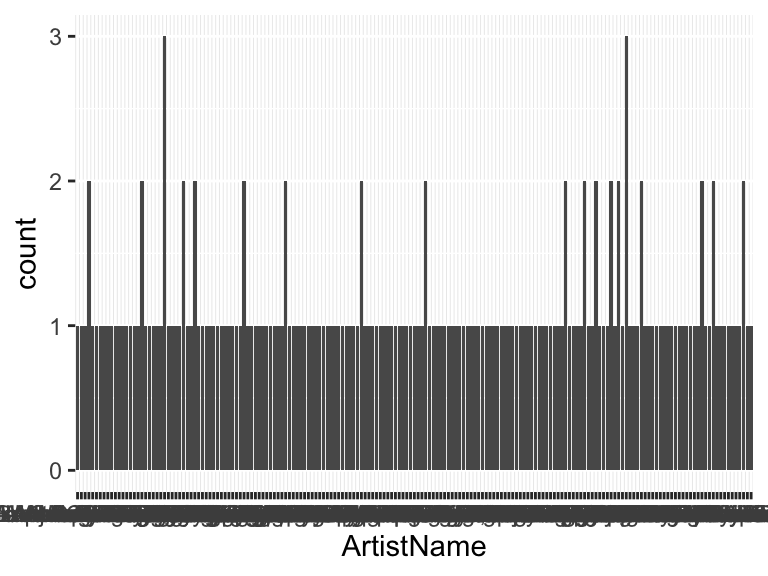• The following values appear with prefixed or suffixed white space: "Giuseppe Arcimboldo ".

• Note that the following levels have at most five observations: "Adolph von Menzel", "Alberto Giacometti", "Albrecht Altdorfer", "Albrecht Durer", "Alexej von Jawlensky", …, "William Holman Hunt", "William McTaggart", "William Turner", "Winslow Homer", "Wolf Vostell" (169 values omitted).

## NoOfMiddlenames

• Note that this variable is treated as a factor variable below, as it only takes a few unique values.
Feature Result
Variable type numeric
Number of missing obs. 0 (0 %)
Number of unique values 4
Mode “0”
Reference category 0
No. zeros 157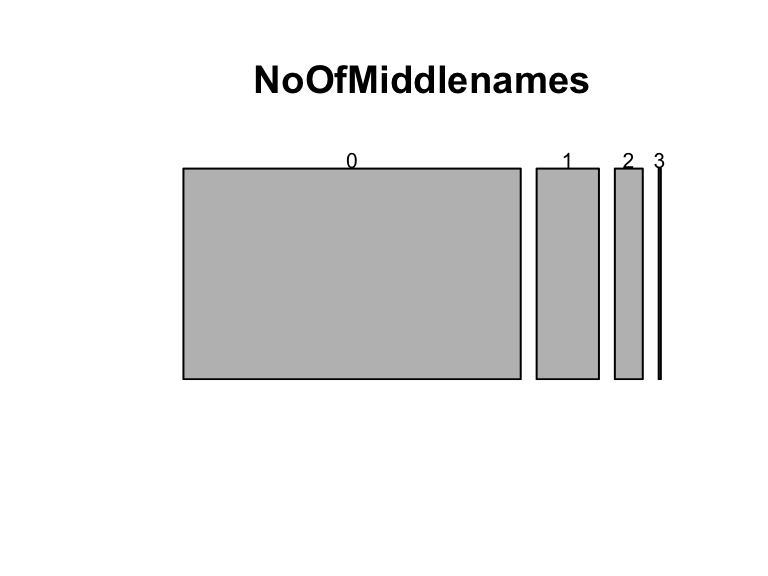• Note that the following levels have at most five observations: "3".

## Title

• The variable is a key (distinct values for each observation).

## Year

Feature Result
Variable type integer
Number of missing obs. 0 (0 %)
Number of unique values 149
Median 1851.5
1st and 3rd quartiles 1627.75; 1914
Min. and max. 1150; 1968
No. zeros 0
Mean 1765.73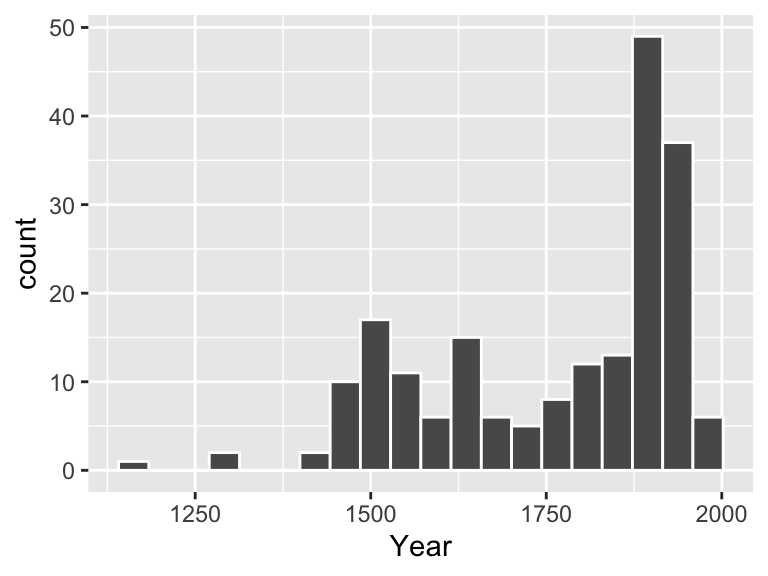• Note that the following possible outlier values were detected: "1958", "1961", "1965", "1968".

## Location

Feature Result
Variable type character
Number of missing obs. 3 (1.5 %)
Number of unique values 97
Mode “National Gallery”
No. zeros 0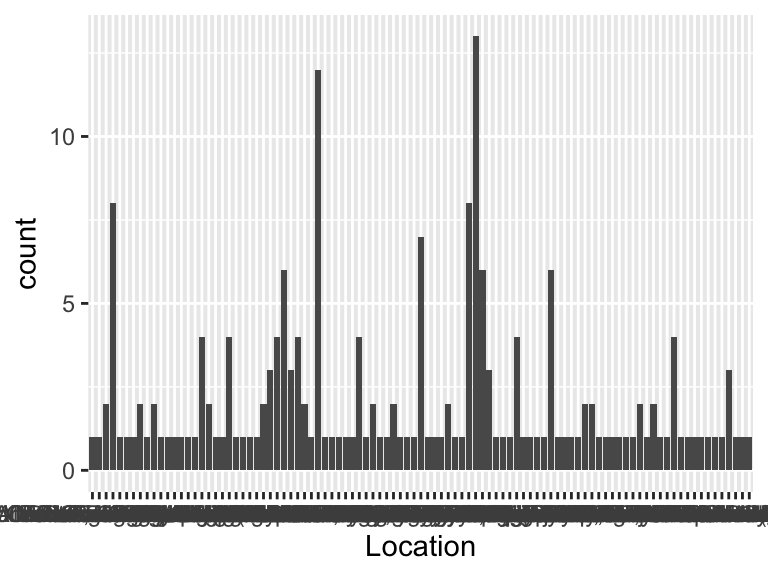• Note that the following levels have at most five observations: "Alfred Stieglitz Collection, Carl Van Vechten Gallery", "Alte Nationalgalerie", "Alte Pinakothek", "Brancacci Chapel", "Centre Pompidou", …, "von Zezschwitz (for sale)", "Wallraf-Richartz Museum", "Walters Art Museum", "Whitney Museum of American Art", "Wildenstein Collection" (79 values omitted).

## Continent

Feature Result
Variable type factor
Number of missing obs. 0 (0 %)
Number of unique values 3
Mode “Europe”
Reference category Asia
No. zeros 0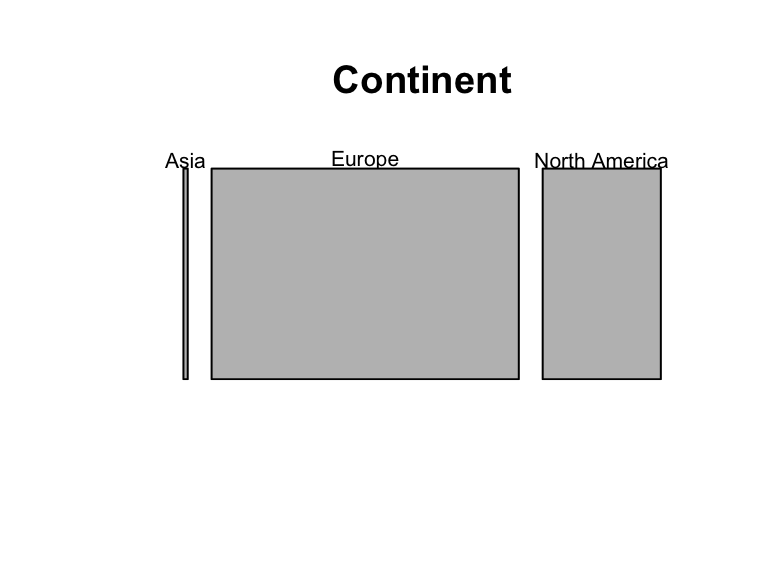• Note that the following levels have at most five observations: "Asia".

## Width

Feature Result
Variable type numeric
Number of missing obs. 10 (5 %)
Number of unique values 163
Median 122.45
1st and 3rd quartiles 77; 198.3
Min. and max. 10.7; 990
No. zeros 0
Mean 168.46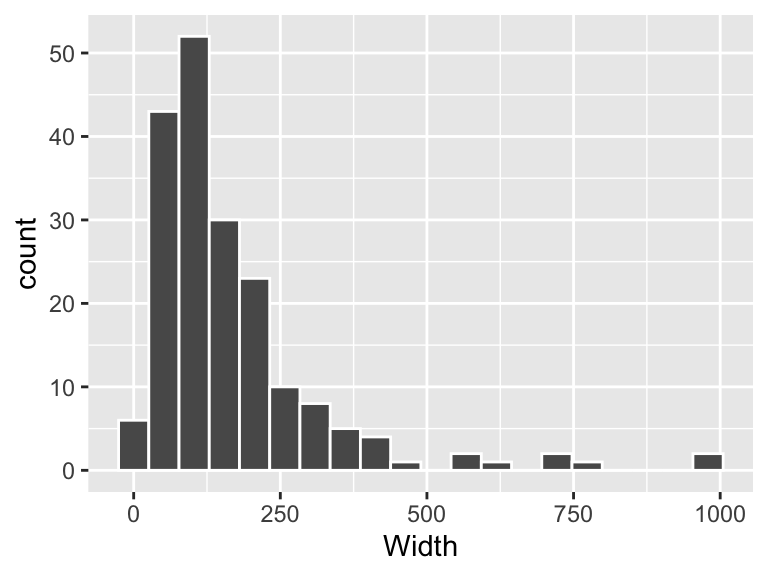• Note that the following possible outlier values were detected: "10.7", "13.9", "14.7", "15.2", "17.2", …, "28", "32", "776", "957", "990" (3 values omitted).

## Height

Feature Result
Variable type numeric
Number of missing obs. 10 (5 %)
Number of unique values 164
Median 113
1st and 3rd quartiles 73.12; 168.75
Min. and max. 12.3; 666
No. zeros 0
Mean 134.51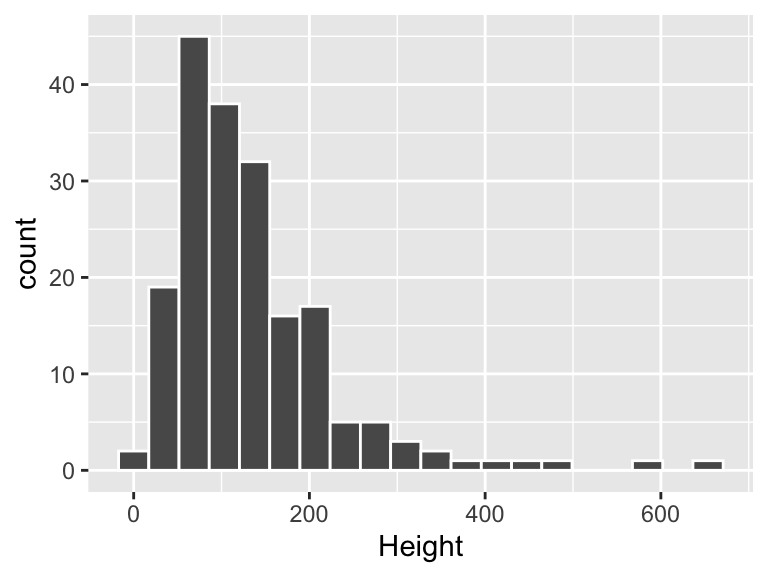• Note that the following possible outlier values were detected: "460", "491", "585", "666".

## Media

Feature Result
Variable type character
Number of missing obs. 10 (5 %)
Number of unique values 27
Mode “oil paint”
No. zeros 0